# Samacheer Kalvi 10th Maths Solutions Chapter 4 Geometry Ex 4.3

## Tamilnadu Samacheer Kalvi 10th Maths Solutions Chapter 4 Geometry Ex 4.3

Question 1.
A man goes 18 m due east and then 24 m due north. Find the distance of his current position from the starting point?
Solution:
Using Pythagoras theorem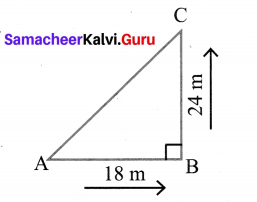AC2 = AB2 + BC2
= (18)2 + (24)2
= 324 + 576
= 900
AC = $$\sqrt{900}$$ = 30 m
∴ The distance from the starting point is 30 m.

Question 2.
There are two paths that one can choose to go from Sarah’s house to James house. One way is to take C street, and the other way requires to take A street and then B street. How much shorter is the direct path along C street? (Using figure).Solution:
By using Pythagoras theorem
AC2 = AB2 + BC2
= 22 + (1.5)2
= 4 + 2.25
= 6.25
AC = 2.5 miles.
If one chooses C street the distance from James house to Sarah’s house is 2.5 miles
If one chooses A street and B street he has to go 2 + 1.5 = 3.5 miles.
2.5 < 3.5, 3.5 – 2.5 = 1 Through C street is shorter by 1.0 miles.
∴ The direct path along C street is shorter by 1 mile.To round to the nearest tenth, look at the tenth’s place (right after the decimal).

Question 3.
To get from point A to point B you must avoid walking through a pond. You must walk 34 m south and 41 m east. To the nearest meter, how many meters would be saved if it were possible to make a way through the pond?
Solution:
By using Pythagoras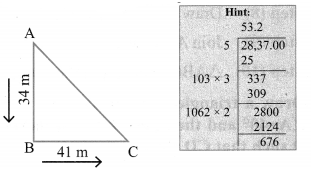AC2 = AB2 + BC2
= 342 + 412
= 1156+ 1681
= 2837
AC = 53.26 m
Through B one must walk 34 + 41 = 75 m walking through a pond one must comes only 53.2 m
∴ The difference is (75 – 53.26) m = 21.74 m
∴ To the nearest, one can save 21.74 m.

Question 4.
In the rectangle WXYZ, XY + YZ = 17 cm, and XZ + YW = 26 cm. Calculate the length and breadth of the rectangle?Solution:
XY + YZ = 17 cm …………. (1)
XZ + YW = 26 cm ………… (2)
(2) ⇒ XZ = 13, YW = 13
(∵ In rectangle diagonals are equal).
(1) ⇒ XY = 5, YZ = 12 XY + YZ = 17
⇒ Using Pythagoras theorem
52 + 122 = 25 + 144 = 169 = 132
∴ In ∆XYZ = 132 = 52 + 122 it is verified
∴ The length is 12 cm and the breadth is 5 cm.Question 5.
The hypotenuse of a right triangle is 6 m more than twice of the shortest side. If the third side is 2 m less than the hypotenuse, find the sides of the triangle?
Solution:
Let a is the shortest side.
c is the hypotenuse
b is the third side.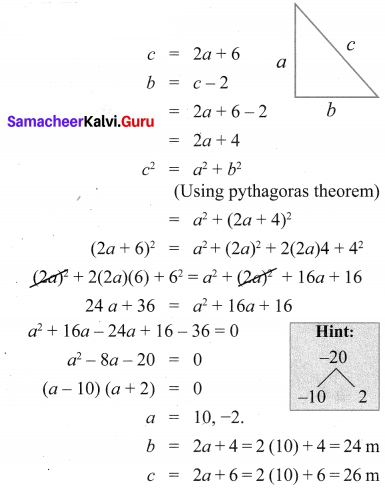∴ The sides of the triangle are 10m, 24m, 26m.
Verification 262 = 102 + 242
676 = 100 + 576 = 676

Question 6.
5 m long ladder is placed leaning towards a vertical wall such that it reaches the wall at a point 4m high. If the foot of the ladder is moved 1.6 m towards the wall, then find the distance by which the top of the ladder would slide upwards on the wall.
Solution:
Let the distance by which top of the slide moves upwards be assumed as ‘x’.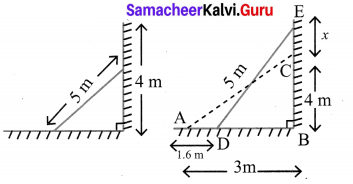From the diagram, DB = AB – AD
= 3 – 1.6 ⇒ DB = 1.4 m
also BE = BC + CE
= 4 + x
∴ DBE is a right angled triangle
DB2 + BE2 = DE2 ⇒ (1.4)2 + (4 + x)2= 52
⇒ (4 + x)2 = 25 – 1.96 ⇒ (4 + x)2 = 23.04
⇒ 4 + x = $$\sqrt{23.04}$$ = 4.8
⇒ x = 4.8 – 4 ⇒ x = 0.8 mQuestion 7.
The perpendicular PS on the base QR of ∆PQR intersects QR at S, such that QS = 3 SR. Prove that 2PQ2 = 2PR2 + QR2.
Solution: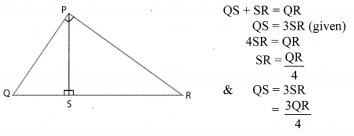In ∆PQS,
PQ2 = PS2 + QS2 ………… (1)
In ∆PSR,
PR2 = PS2 + SR2 ……….. (2)
(1) – (2) ⇒ PQ2 – PR2 = QS2 – SR2 …………. (3)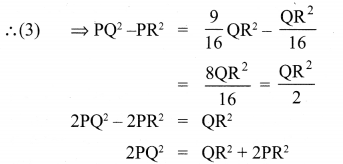Hence it proved.

Question 8.
In the adjacent figure, ABC is a right-angled triangle with right angle at B and points D, E trisect BC. Prove that 8AE2 = 3AC2 + 5AD2.Solution:
Since D and E are the points of trisection of BC,
therefore BD = DE = CE
Let BD = DE = CE = x
Then BE = 2x and BC = 3x
In right triangles ABD, ABE and ABC, (using Pythagoras theorem)
We have AD2 = AB2 + BD2
⇒ AD2 = AB2 + x2 ……………. (1)
AE2 = AB2 + BE2
⇒ AB2 + (2x)2
⇒ AE2 = AB2 + 4x2 ………… (2)
and AC2 = AB2 + BC2 = AB2 + (3x)2
AC2 = AB2 + 9x2
Now 8 AE2 – 3 AC2 – 5 AD2 = 8 (AB2 + 4x2) – 3 (AB2 + 9x2) – 5 (AB2 + x2)
= 8AB2 + 32x2 – 3AB2 – 27x2 – 5AB2 – 5x2
= 0
∴ 8 AE2 – 3 AC2 – 5 AD2 = 0
8 AE2 = 3 AC2 + 5 AD2.
Hence it is proved.